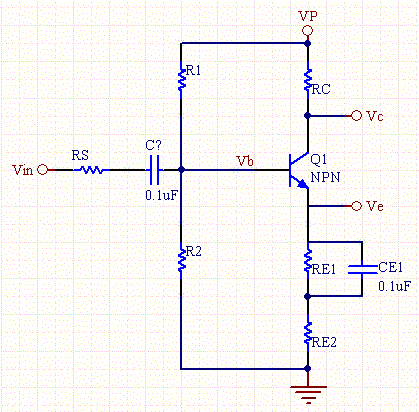# BJT Transistor as a Switch, Saturation Calculator

The following calculators, will compute all of the bias values of the transistor circuit, given the supply voltage, and the base voltage, and all of the resistor values. The beta and Vd transistor parameters, can be measured, or gathered from a data sheet. If unknown, the default values below can be used, since the circuit is normally fairly insensitive to these values.

This calculator also determines if the transistor is in saturation or cut off, the frequency response, and internal resistive and capacitive parameters for both the CE (common emitter) and CC (common collector, also known as emitter follower) configurations.Transistor Biased with voltage dividerTransistor Biased with series resistor

 Base Bias Type Series Resistor Voltage Divider RB (Base resistor) (K ohms) VB (Bias Voltage) (V) R1 (K ohms) R2 (K ohms) RC (Collector resistor) (K ohms) RE1 (Emitter resistor 1) Bypassed (K ohms) RE2 (Emitter resistor 2) Gain control (K ohms) VP (Supply Voltage) (V) Beta (DC Current Gain) VBE (Base to emitter drop) (V) Rs (Source Resistance): (ohms) RL (Load resistor) (ohms) fT (Current Gain BW Product): Optional (MHz) CCB (Collector-Base Cap.): Optional (pF) CBE (Base-Emitter Cap.): Optional (pF) Vc (Collector Voltage) (V) Ve (Emitter Voltage) (V) Vb (Base Voltage) (V) Ic (Collector Current) (mA) Ib (Base Current) (mA) gm (Transconductance) rπ (Input Resistance of BJT at low freq) Saturated Cut off region CE (Common Emitter) Configuration Parameters A (Amplifier Voltage Gain) Rin (ohms) CMCE (Miller Capacitance) (pF) BWCE (BW) (MHz) RO ohms CC (Common Collector) Configuration Parameters A (Amplifier Voltage Gain) Rin (ohms) BWCC (BW) (MHz) RO ohms

DC biasing of a transistor is one of the most common electrical engineering tasks. Transistors need certain DC levels for them to function correctly. These DC are also know as their bias point. Any AC signals which are injected into a transistor circuit ride on top of these DC signals. Because of the principle of linearity, the DC bias point and AC signals are design independently. Another way of looking at bias is to measure what are the DC values are at the various nodes within the circuit.

Depending upon how the transistor is biased it can act as a switch or an amplifier, or buffer. When the transistor is biased as a switch, resistor RE is set to zero ohms (shorted out of the circuit), and the base voltage is set to a level which saturates the transistor (turns it fully on).

For amplifiers, the input signal is usually AC coupled through a capacitor to the bias resistor.

The common emitter configuration (class A amp), is the most common type of amplifier transistor amplifier. The input signal is injected into the base through a coupling capacitor, and the output is taken off the transistor collector. The output signal is an amplified and inverted version of the input signal. The output signal typically can't swing the full range, because as the collector current goes too high the transistor saturates, with the signal swinging toward ground. Typically the base bias point is set to be about 1/3 of the collector voltage. The emitter resistor is typically bypassed with a large capacitor, so that it looks like a short to AC signals, otherwise it reduces the gain of the AC signal.

The emitter follower is a buffer circuit, which gives a gain close to 1. It is used because it has high input resistance, and can provide good drive.

You may also fill in frequency information for the transistor and it's nominal frequency operation point, and the calculator will compute frequency dependent parameters, and the first pole which limits the bandwidth of the amplifier.

#### Equations

Ib*Rb+Ib*Beta*Re+Vbe = Vin

Ib= (Vin-Vbe)/(Rb+Beta*Re);

Ic= Beta*Ib;

Ve= Ic*Re;

Vb= Ve+Vbe;

Vc= VP - Ic*Re;

If Vc<Ve the transistor is saturated.

if Vin< Vb the transistor is in cut off mode.

gm = Ic/25mA

rπ= Beta/gm

fT= gm/(2π*(CBE+CBC))

For the Common Collector:

RO= RE || [re+(RB||RS)/(Beta+1)]

Rin= R1||R2||(rπ+(Beta+1)*RE2)

For the Common Emitter:

Ro= ~Rc;

Rin= R1||R2||(rπ+(Beta+1)*RE2)